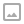## 「HAOI2016」找相同字符-后缀数组+单调栈

·  ✏️ About  1661 words  ·  ☕ 4 mins read · 👀... views

Luogu P3181

## 题解

### 解法四：$O(n \log n)$

s_3 = s_1 + "?" + s_2 ，那么答案就是 cal(s3)-cal(s1)-cal(s2) ，其中 ? 是一个没有在字符串里面出现的字符。

## 代码

  1 2 3 4 5 6 7 8 9 10 11 12 13 14 15 16 17 18 19 20 21 22 23 24 25 26 27 28 29 30 31 32 33 34 35 36 37 38 39 40 41 42 43 44 45 46 47 48 49 50 51 52 53 54 55 56 57 58 59 60 61 62 63 64 65 66 67 68 69 70 71 72 73 74 75 76 77 78 79 80 81 82 83 84 85 86 87 88 89 90 91 92 93 94 95 96 97 98 99 100 101 102 103 104 105 106 107 108 109 110 111  #include #define ll long long #define pp pair using namespace std; const int MAXN = 233333; template struct SA{ int s[siz<<1],p[siz],t[siz<<1]; int sa[siz],rk[siz],ht[siz]; int b[siz],cur[siz]; #define pushL(x) sa[cur[s[x]]++] = x #define pushS(x) sa[cur[s[x]]--] = x #define inducedSort(v)\ fill_n(b,m,0),fill_n(sa,n,-1);\ for(int i = 0;i0&&t[sa[i]-1]) pushL(sa[i]-1);\ for(int i = 0;i0&&!t[sa[i]-1]) pushS(sa[i]-1); void sais(int n,int m,int *s,int *t,int *p){ int ch = rk = -1,n1 = t[n-1] = 0,*s1 = s+n; for(int i = n-2;~i;--i) t[i] = s[i]==s[i+1]?t[i+1]:s[i]>s[i+1]; for(int i = 1;i int mapp(const T *str,int n){ int m = *max_element(str,str+n); fill_n(rk,m+1,0); for(int i = 0;i void SuffixArray(const T *str,int n){ int m = mapp(str,++n); sais(n,m,s,t,p); } void getheight(int n){ for(int i = 0;i<=n;i++) rk[sa[i]] = i; for(int i = 0,h=ht=0;i<=n;i++){ int j = sa[rk[i]-1]; while(i+h void build(const T *str,int n){ SuffixArray(str,n); getheight(n); } }; char s1[MAXN],s2[MAXN],s3[MAXN<<1]; void init(){ scanf("%s",s1); scanf("%s",s2); } ll cal(char *a){ int n = strlen(a); a[n] = 'A'-1; static SA<401000> T; T.build(a,n); ll ans = 0,tmp = 0; stack S; for(int i = 1;i<=n;i++){ ll cnt = 1,tot = 0; while(!S.empty() && S.top().first >= T.ht[i]){ cnt += S.top().second; tot += 1LL * S.top().first * S.top().second; S.pop(); } S.push(make_pair(T.ht[i],int(cnt))); tmp += T.ht[i]*cnt - tot; ans += tmp; } return ans; } void solve(){ int n = strlen(s1),m = strlen(s2); memcpy(s3,s1,n),memcpy(s3+n+1,s2,m);s3[n] = 'A'; printf("%lld\n",cal(s3)-cal(s1)-cal(s2)); } signed main(){ init(); solve(); return 0; }WRITTEN BY
cqqqwq
A student in Computer Science.